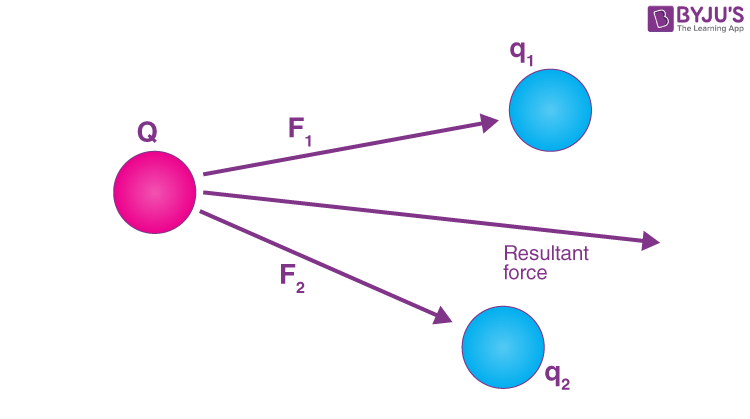# Superposition Principle and Continuous Charge Distribution

## What is the Principle of Superposition?

Superposition Principle lets us calculate the total force on a given charge due to any number of point charges acting on it. Every charged particle creates an electric field in the universe in the space surrounding it. The electric field created due to the charge is independent of the presence or absence of all other charges. The electric field created can be calculated with the help of Coulomb’s law. The principle of superposition allows for the combination of two or more electric fields.

 “The principle of superposition states that every charge in space creates an electric field at point independent of the presence of other charges in that medium. The resultant electric field is a vector sum of the electric field due to individual charges.”

The superposition principle is used to compute the net flux, net field, the net potential energy of the system.

In the next section, let us discuss how the superposition principle is applied in electrostatics.

## Principle of Superposition in Electrostatics

The superposition principle is helpful when there are a large number of charges in a system. Let’s consider the following case,For our convenience let us consider one positive charge, and two negative charges exerting a force on it, from the superposition theorem we know that the resultant force is the vector sum of all the forces acting on the body, therefore the force Fr , the resultant force can be given as follows,

$$\begin{array}{l} \overrightarrow{F_r} ~=~ \frac {1}{4 \pi \in} \left[ \frac {Q q_1}{r^2_{12}} \hat{r}_{12} ~+~\frac {Qq_2}{r^2_{13}} \hat{r}_{13} \right] \end{array}$$

Where,

$$\begin{array}{l} \hat{r}_{12} \,\, and \,\, \hat{r}_{13}\end{array}$$
are the unit vectors along the direction of q1 and q2.

ε is the permittivity constant for the medium in which the charges are placed in.

Q, q1, and q2 are the magnitudes of the charges respectively.

r12  and r13 are the distances between the charges Q and q1  &  Q and q2 respectively.

## Continuous Charge Distribution:

We know that the smallest form of charge we can obtain would be +e or –e i.e. the charge of an electron or a proton, hence charges are quantized. Continuous charge distribution means that all charges are closely bound together having very less space between each other.

There are different ways in which charges can be distributed:

1. Linear charge distribution.
2. Surface charge distribution.
3. Volume charge distribution.

### Linear charge distribution:

The linear charge distribution is when the charges get distributed uniformly along a length, like around the circumference of a circle or along a straight wire, linear charge distribution is denoted by the symbol λ.

λ = dq/dl and it is measured in Coulombs per meter.

### Surface charge distribution:

When a charge is distributed over a specific area, like the surface of a disk, it is called a surface charge distribution, it is denoted by the Greek letter σ.

The surface charge distribution is measured Coulombs per square meter or Cm-2.

### Volume charge distribution:

When a charge is distributed uniformly over a volume it is said to be volume charge distribution, like distribution of charge inside a sphere, or a cylinder. It is denoted by ρ.

The volume charge distribution is measured in coulombs per cubic meter or Cm-3.

Watch the video and understand the principle of superposition clearly with the help of visualisations.Stay tuned with BYJU’S to know more about continuous charge distribution, electrostatics, and much more.

Test your knowledge on Superposition Principle And Continuous Charge Distribution